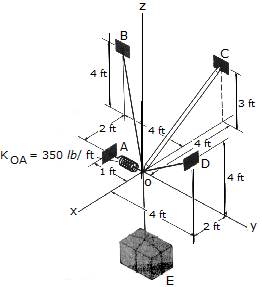# Engineering Mechanics - Equilibrium of a Particle - Discussion

### Discussion :: Equilibrium of a Particle - General Questions (Q.No.5)

5.Determine the tension developed in cables OD and OB and the strut OC, required to support the 500-lb crate. The spring OA has an unstretched length of 0.2 ft and a stiffness of kOA = 350lb/ft. The force in the strut acts along the axis of the strut.

 [A]. Fob = 289 lb, Foc = 175.0 lb, Fod = 131.3 lb [B]. Fob = 86.2 lb, Foc = 175.0 lb, Fod = 506 lb [C]. Fob = 375 lb, Foc = 0, Fod = 375 lb [D]. Fob = 664 lb, Foc = 175.0 lb, Fod = 244 lb

Explanation:

No answer description available for this question.

 Bhaskar said: (Jun 10, 2012) I can't understsnd this prob.

 Sruthi said: (Jan 17, 2015) Will you please tell me the solution?

 Michael Gyan said: (Oct 1, 2016) How is it solve? Please tell me.

 Winfred said: (Nov 13, 2016) First find position coordinates for all points, position vectors, unit vectors, force vectors, equation of equilibrium and solve the resulting equation simultaneously.

 Pramod said: (Dec 14, 2016) Can anybody solve this problem?

 Gideon Odame said: (Sep 18, 2018) I didn't understand. Please explain.